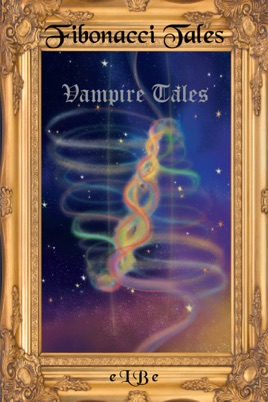• 12,99 €

Beschreibung des Verlags

Fibonacci Tales are fiction written in the format of the Fibonacci sequence, hence the name Fibonacci Tales. What Fibonacci did is plug a 0 and a 1 and set the rule to always add the next two numbers. Each Fibonacci Tales book has:
two one-page chapters,
one two-page chapter,
one three-page chapter,
one five-page chapter,
one eight-page chapter,
one thirteen-page chapter,
one twenty-one-page chapter,
one thirty-four-page chapter,
one fifty-five-page chapter,
and one eighty-nine-page chapter for a total of 232 pages per book.

Fibonacci Tales are written for all ages and in paired sets of books. The first pair of Fibonacci Tales books are Fibonacci Tales Vampire Tales and Fibonacci Tales Knights Tales.

The second pair of Fibonacci Tales books are Fibonacci Tales Dust Tales and Fibonacci Tales Mother Tales.

The third pare of Fibonacci Tales books will be called Fibonacci Tales Cat Tales and Fibonacci Tales Goddess Tales. These books are works in progress during mid-September 2016. The author expects to complete the third pair of Fibonacci Tales books and available around early to mid-2017.

Fibonacci Tales books are designed for electronic book reading. Each pair of books includes music callouts that are essential to the stories (music has the power to calm the savage beast), and therefore, Fibonacci Tales books do not lend themselves to printed book format.

Plus the cost of printing two pairs of books and pressing two CDs for each pair of books would not be cost-effective, and it would be an outright irritant for the dear reader who would have to advance the CD one tune at a time and stop the CD before the next tune began as CD players are designed to do. I would utterly hate to read Fibonacci Tales in printed book format. For that reason alone, scratch the idea of publishing Fibonacci Tales in physical book format. I will not agree.

On the plus side again, I have two disinterested readers who will read and comment on Fibonacci Tales books once they are available in e-book format.